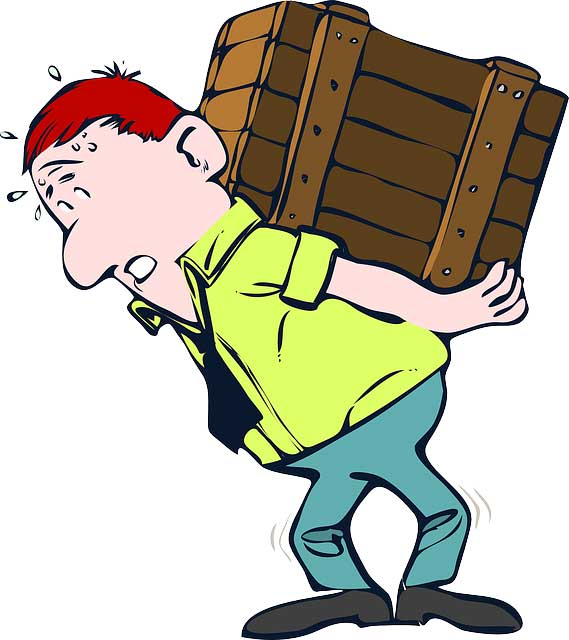## What is Force?

Everything you see around yourself is surrounded by forces. These force may be push-pulls or gravitational. Forces can accelerate, slow-down, and change the shape of an object. So, what is the definition of the force? Force is actually an interaction which when applied to an object, changes or tends change it state of motion or rest. In other words, force can bring the objects that are not moving into motion. Similarly, it can bring the moving objects into the full stop.

Force is a vector quantity, that means it requires magnitude along with a direction in which the force is applied.

## How is Force Calculated?

Force is measured in newton, abbreviated as “N”. One newton is equal to a force, which when applied to an object having a weight of 1 kg, accelerates it to 1 m/s^2. That means, if you constantly apply one newton force to a 1 kg heavy object, its velocity will increase by 1 meter per second in each passing second until you remove the force.

Force is calculated by using the Newton’s second law of motion:

F = m*a

Here “m” is the mass of the object and “a” is the acceleration of that object.

Example: A bike has a mass of 100 kg which is accelerating with an acceleration of 5 m/s^2. Calculate the force that is acting upon it.

Solution:

You are given the mass of the bike and acceleration of the bike. Just apply the Newton’s second law of motion.

F = m*a = 100*5 N = 500 N

## Types of Force

There are various types of force, according to their nature. Few most familiar types of force are:

1. Friction: It is the force which opposes the motion of an object. When you kick a box, it will come to rest at a certain It is the friction that is slowing down the box. Friction only acts on the moving objects.
2. Gravitation: Gravitation is an attractive force that is caused by heavy objects, like planets. This force attracts anything that has mass. You are standing on the Earth due to the gravitational force of the Earth.
3. Tension: It is the pulling force which is exerted on strings, cables or any similar object like them.

## Facts

• Torque is kind of force that rotate or twist the objects.
• Gravitational and electromagnetic forces don’t need to have a contact with the object for exerting force. They can exert a force on objects which are away from them.
• Force was described by the Isaac Newton in his law called Newton’s second law of motion.